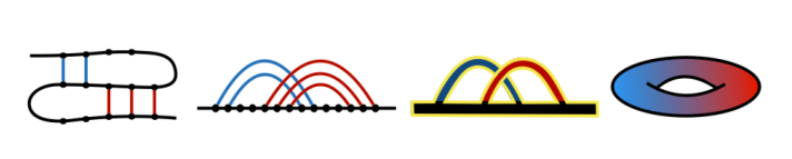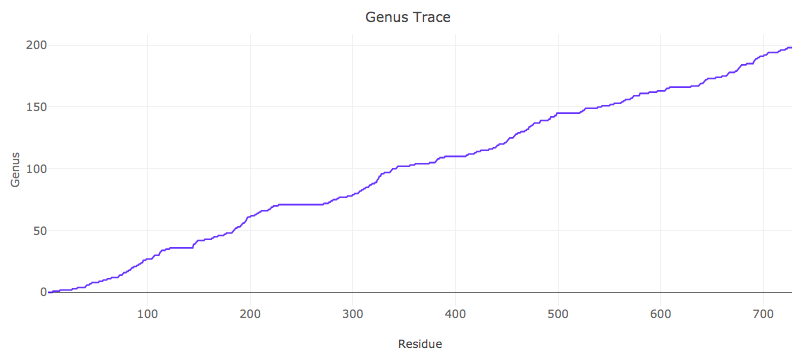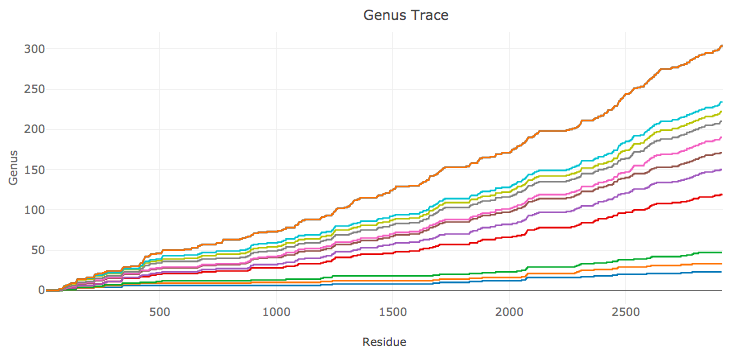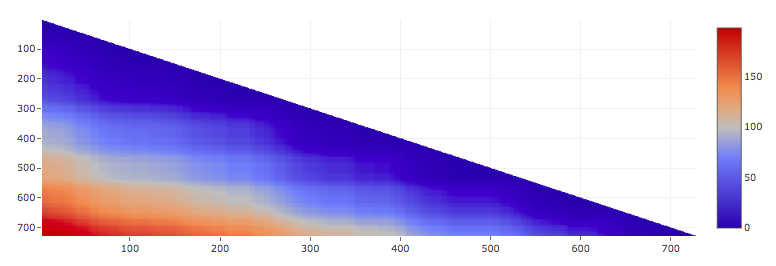#### Genus for Biomolecules - About

The “Genus for Biomolecules” database collects information about topological structure and complexity of proteins and RNA chains, which is captured by the genus of a given chain and its subchains. For each biomolecule this information is shown in the form of a genus trace plot, as well as a genus matrix diagram. We assemble such information for all protein and RNA structures deposited in the PDB. This database presents also various statistics and extensive information about biological function of analyzed biomolecules. Moreover, users can analyze their own structures.

The genus trace and genus matrix capture various physical and biological information about biomolecules and their structural complexity . The genus analysis enables to quantify how much more complicated a biomolecule is than it would be suggested by its nested secondary structure. For multi-domain proteins, the genus trace detects their domain structure. The genus analysis can be also conducted for complexes, and beyond the realm of biomolecules, with various other applications.

Structure of bonds in a biomolecule (e.g. canonical and non-canonical pairs in RNA, or contacts in proteins) can be encoded in the form of a chord diagram. On the other hand, it is known that each chord diagram encodes information about some two-dimensional surface. The genus provides basic topological characteristics of such a surface – it is equal to the number of “holes” in such a surface (for example sphere has the genus g=0, and for doughnut g=1), and it can be deduced from the form of the corresponding chord diagram. Therefore, it follows that to each biomolecular chain one can associate a number – the genus of the corresponding auxiliary surface, which is also encoded in a chord diagram that represents structure of bonds in this chain. The genus is larger if structure of bonds is more complicated, i.e. if there are more urations analogous to pseudoknots in RNA. However, simply increasing the number of bonds (without pseudoknot-like structure) does not change the genus. In this sense the genus is the measure of complexity of bonds.

The relation between a pattern of bonds in a biomolecules, the corresponding chord diagram, and an auxiliary surface, is shown in Fig. 1. Suppose we consider a biomolecule that consists of b chains (also called backbones; in this example b=1), and n bonds. Such a structure (left panel) can be presented in the standard way as a chord diagram (second-to-left panel), in which all backbones are represented as horizontal intervals, and bonds connecting pairs of residues are represented by arcs. Parallel chords do not affect the value of the genus – therefore all sets of parallel bonds can be replaced by a single bond (second-to right panel) (this in particular shows that the genus depends on complexity and crossing between bonds, and not just their number). One can now replace a chord diagram by its thickened version, and count the number r of its boundaries (in red, r=1 in this example). Such a thickened diagram can be drawn on a two-dimensional surface of a unique genus g, which can be computed using the Euler formula

b – n = 2 – 2g – r.

In the example in Fig. 1 we have b=1, n=2, and r=1, so that the above formula indeed implies, g=1 as expected.Fig. 1. Relation between a patterns of bonds (left panel), chord diagram (second-to-left panel) and its thickened version (second-to-right panel), and an auxiliary surface (right panel) of a given genus g (g=1 in this example).

The genus of RNA chains was considered one of the first times in [2,3]. In this and a number of subsequent works, the genus of the whole RNA chains was analyzed – i.e. to each RNA structure a single number (its genus g) was assigned. In these works it was also shown that chord diagrams can be related to random matrix theory and so called matrix models. This relation turns out to be quite deep; in particular in  a new matrix model (nowadays called “RNA matrix model”) was constructed, which enables to classify and explicitly enumerate all RNA-like configurations.

Apart from considering the genus of the whole biomolecule (which was the main subject of [2,3] and subsequent works), it turns out that much information is encoded in its various subchains. In  a new notion of the “genus trace” was introduced. The genus trace is a function that captures values of genus for all subchains spanned between the first and any other residue in a given biomolecule – it could be interpreted e.g. as observing how the molecule folds up during synthesis from the point of view of an observer located at the synthesis end. An example of a genus trace plot, for Horse Plasma Gelsolin (PDB code 1d0n), is shown in Fig. 2.Fig. 2. Genus trace for Horse Plasma Gelsolin (PDB code 1d0n).

It is also of interest to consider refined genus traces, which take into account some particular type of bonds. In this database such an analysis is conducted for RNA chains, for which we consider all (including non-canonical) types of base pairs, in the Leontis-Westhof classification . We order these base pairs according to frequency of their occurrence [1,6]:

cWW, tHS, tWH, tSS, cWS, tWS, cHS, tWW, cWH, tHH, cSS, cHH (*)

For each RNA chain we compute genus traces only for cWW base pairs, for all cWW and tHS base pairs, for cWW and tHS and tWH base pairs, etc. (in the order given in (*)). An example of such an analysis is shown in Fig. 3.Fig. 3. Genus traces for RNA structure – a large ribosomal unit from Haloarcula Marismortui (PDB code 4v9f). The bottom plot (in blue) is the genus trace calculated by taking into account only canonical cWW base pairs. Each other consecutive plot includes non-canonical base pairs of an additional type, in the order given in (*).

It is also of interest to consider genus for all subchains of a given biomolecule. Such an information can be presented in a matrix (triangular) form, with the genus of a subchain spanned between i’th and j’th residues shown by a point at location (i,j), shown in appropriate color. An example of a genus matrix, for Horse Plasma Gelsolin (PDB code 1d0n), is shown in Fig. 4.Fig. 4. Genus matrix for Horse Plasma Gelsolin (PDB code 1d0n).

In this database, for each protein and RNA chain, we calculate and present the following data:

• the value of genus for the whole chain,

• genus trace (and in addition, for RNA chains, the whole set of genus traces, in the order given in (*)),

• matrix plot, which is shown in 2-dimensional and 3-dimensional version,

• circular chord diagram,

• we also present JSmol presentation of a given structure, its chain sequence, various information about this structure, and a list of similar chains.

Note that various plots that we present are interactive.

 Zając, S., Geary, C., Andersen, E.S., Dabrowski-Tumanski, P., Sulkowska, JI. & Sułkowski, P.,

Genus trace reveals the topological complexity and domain structure of biomolecules.

Scientific Reports 8, 17537 (2018).

 Vernizzi, G., Orland, H. & Zee, A.,

Enumeration of RNA structures by matrix models.

Phys. Rev. Lett. 94, 168103 (2005).

 Bon, M., Vernizzi, G., Orland, H. & Zee, A.,

Topological classification of RNA structures.

J. Mol. Biol. 379(4), 900 (2008).

 Andersen, J.E., Chekhov, L., Penner, R., Reidys, C. & Sułkowski, P.,

Topological recursion for chord diagrams, RNA complexes, and cells in moduli spaces.

Nucl. Phys. B866, 414 (2013).

 Leontis, N. & Westhof, E. ,

Geometric nomenclature and classification of RNA base pairs.

RNA 7(4), 499 (2001).

. Waleń, T., Chojnowski, G., Gierski, P. & Bujnicki, J.M.,

ClaRNA: a classifier of contacts in RNA 3D structures based on a comparative analysis of various classification schemes.

Nucleic Acids Res. 42(19), e151 (2014).